# 如何成為專家 - 心態篇（用心讓學習變好玩）

## 為什麼我的學習不好玩？

1. 找到對的道路是必須的：於是我就算發現對的做法，也只會覺得這沒什麼，要繼續找到下一個，然後就帶來壓力（會不會下一個就卡關？如果我沒找到正確答案怎麼辦？）。
2. 不想嘗試跟目標沒有太直接相關的東西：如果看起來是錯的方向、或不是跟目標極度相關的東西，我就不願意去試試、玩玩看。（可是，我們的經驗跟智慧是有限的，有時候自以為無關的方向，其實可能很有用，科學史上也有很多無意間發現的成果）``````string intToRoman(int num) {
string res;

for(int i=0; i<4; i++) {
int digit = num%10;
num /= 10;

if(digit == 0) continue;

if(i == 0) {
if(digit <= 3) {
for(int j=0; j<digit; j++)
res += "I";
}
else if(digit == 4) res += "IV";
else if(digit == 5) res += "V";
else if(5< digit && digit <= 8) {
res += "V";
for(int j=0; j<digit-5; j++)
res += "I";
}
else if(digit == 9) res += "IX";
}
else if(i == 1) {
if(digit <= 3) {
for(int j=0; j<digit; j++)
res.insert(0, "X");
}
else if(digit == 4) res.insert(0, "XL");
else if(digit == 5) res.insert(0, "L");
else if(5< digit && digit <= 8) {
res.insert(0, "L");
for(int j=0; j<digit-5; j++)
res.insert(j+1, "X");
}
else if(digit == 9) res.insert(0, "XC");
}
else if(i == 2) {
if(digit <= 3) {
for(int j=0; j<digit; j++)
res.insert(0, "C");
}
else if(digit == 4) res.insert(0, "CD");
else if(digit == 5) res.insert(0, "D");
else if(5< digit && digit <= 8) {
res.insert(0, "D");
for(int j=0; j<digit-5; j++)
res.insert(j+1, "C");
}
else if(digit == 9) res.insert(0, "CM");
}
else if(i == 3) {
for(int j=0; j<digit; j++)
res.insert(0, "M");
}
}

return res;
}
``````

``````string intToRoman(int num) {
vector<string> M = {"", "M", "MM", "MMM"};
vector<string> C = {"", "C", "CC", "CCC", "CD", "D", "DC", "DCC", "DCCC", "CM"};
vector<string> X = {"", "X", "XX", "XXX", "XL", "L", "LX", "LXX", "LXXX", "XC"};
vector<string> I = {"", "I", "II", "III", "IV", "V", "VI", "VII", "VIII", "IX"};
return M[num/1000] + C[(num%1000)/100] + X[(num%100)/10] + I[num%10];
}
``````## 好玩學習的充分條件一 - 給自己時間，不逼自己找到正確答案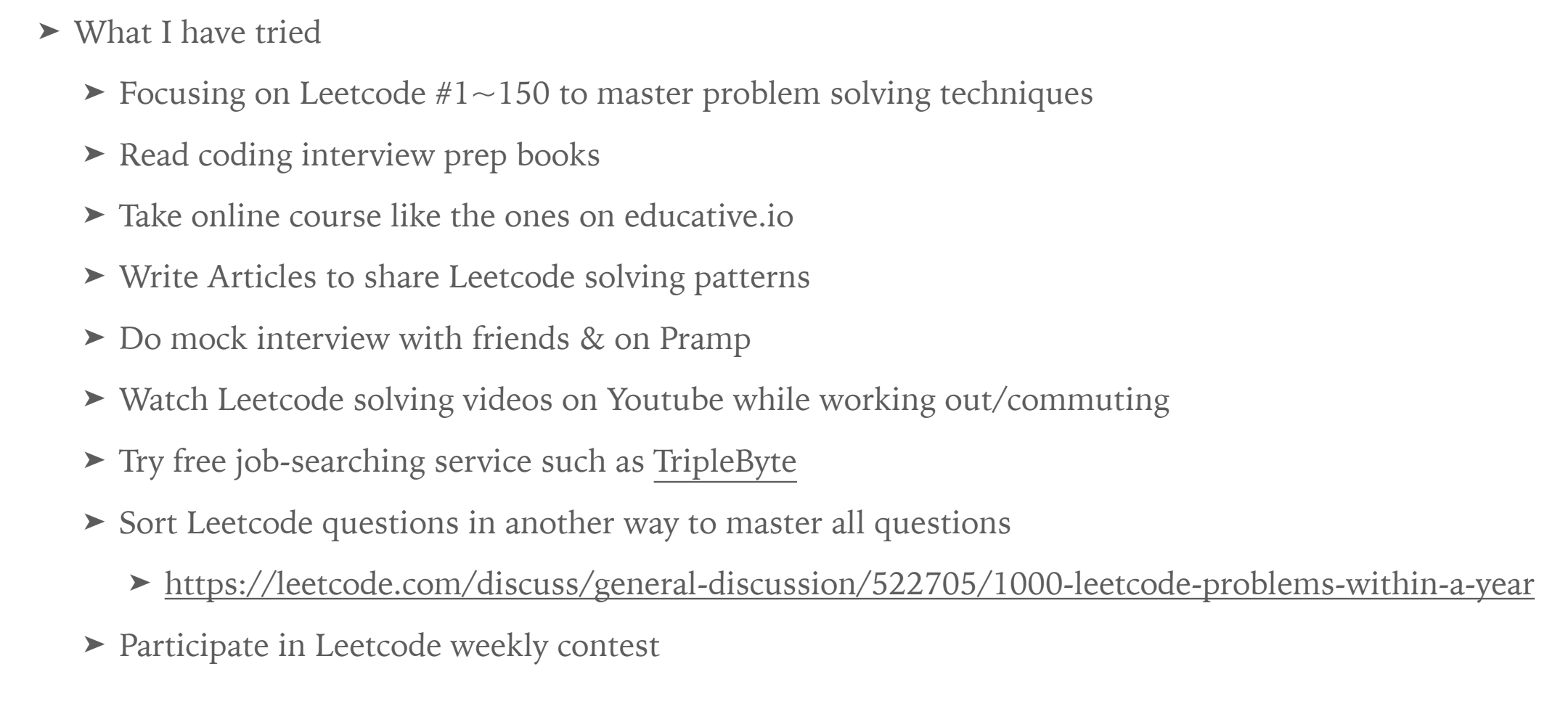## 好玩學習的充分條件二 - 感受每一小步進展的意義## 好玩學習的充分條件三 - 常常關注自己的狀態，已經在勉強學習就先停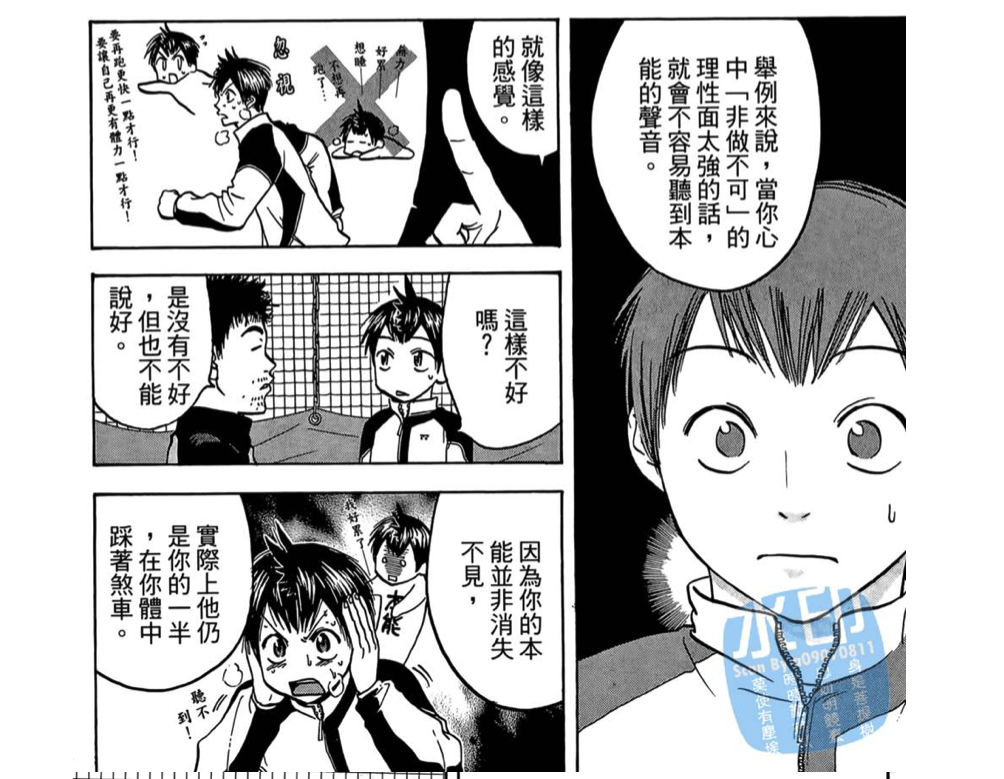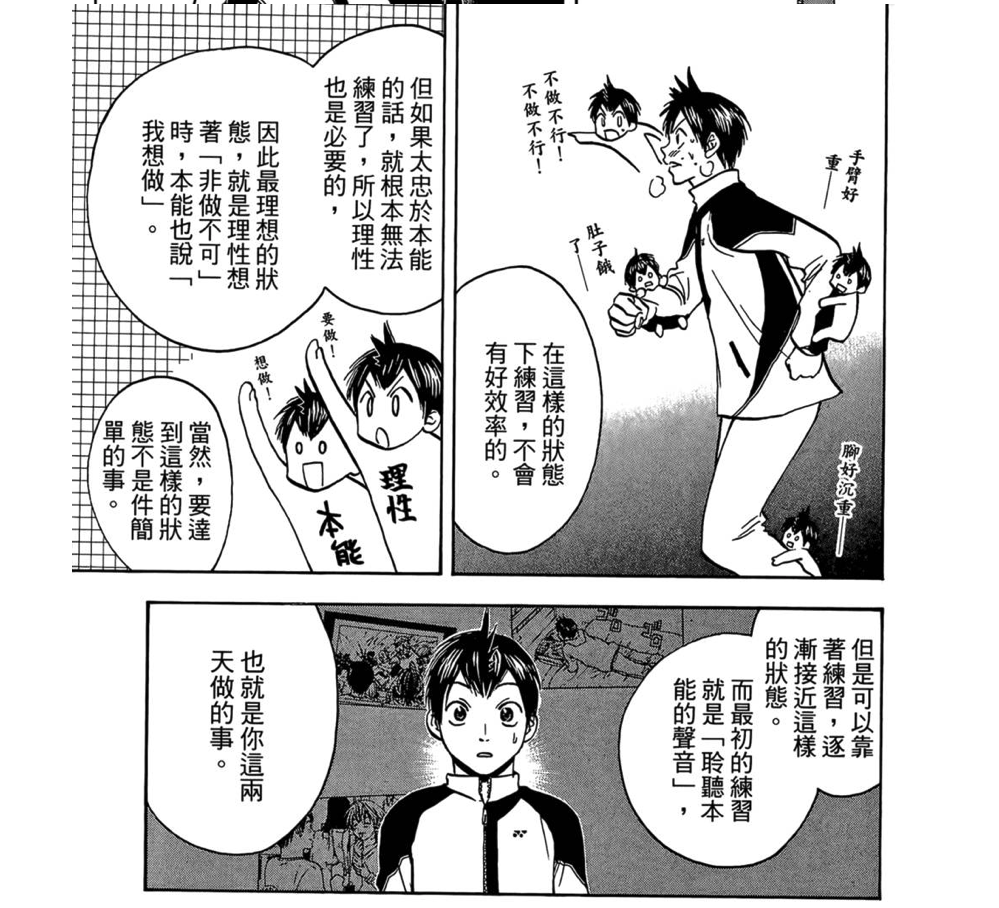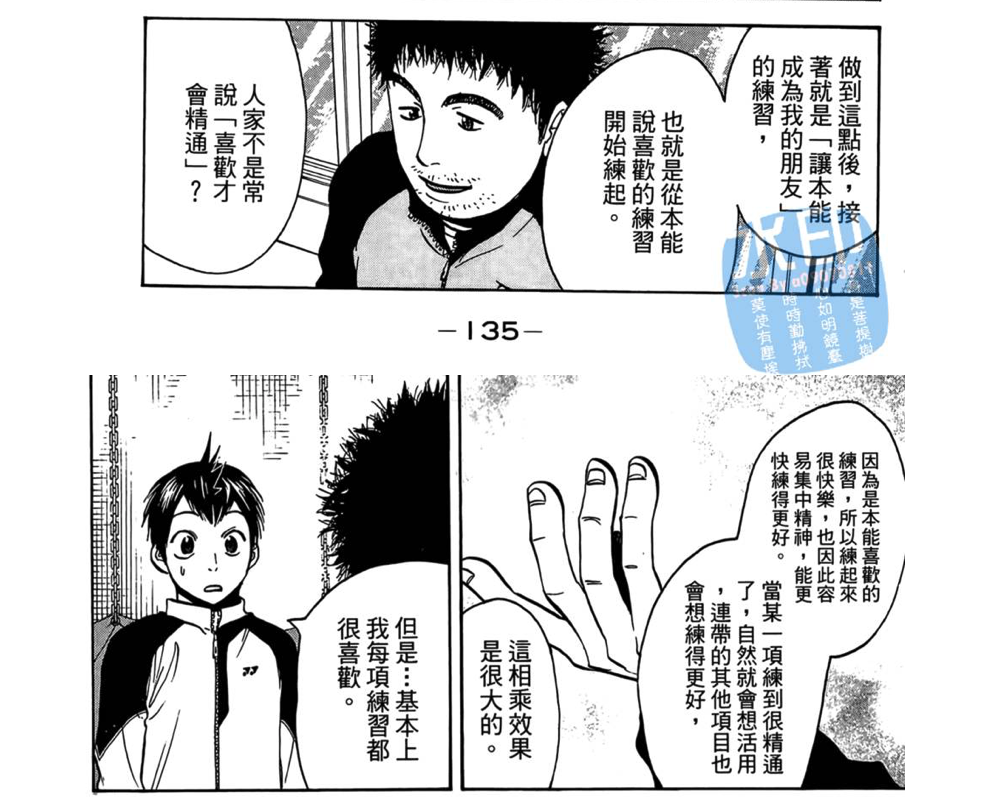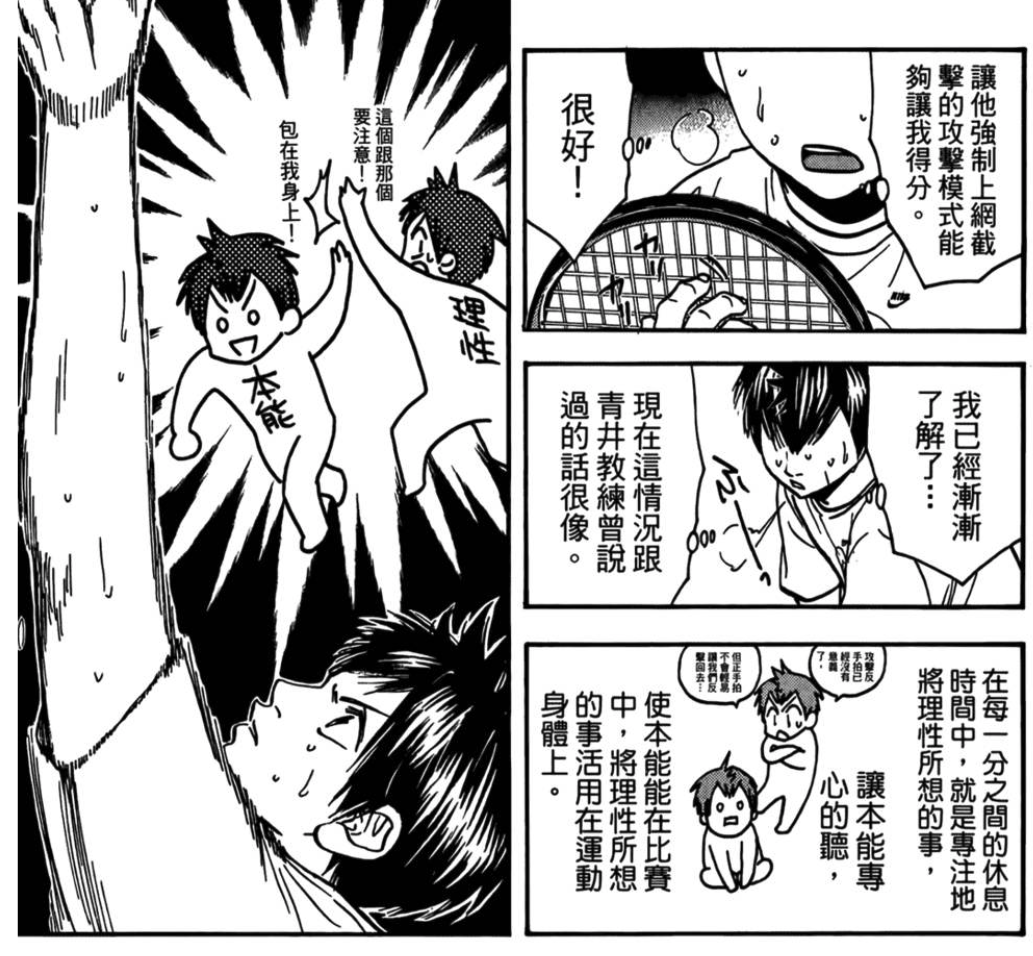## 比達成目標更重要的事 - 建立好的學習系統（or 習慣）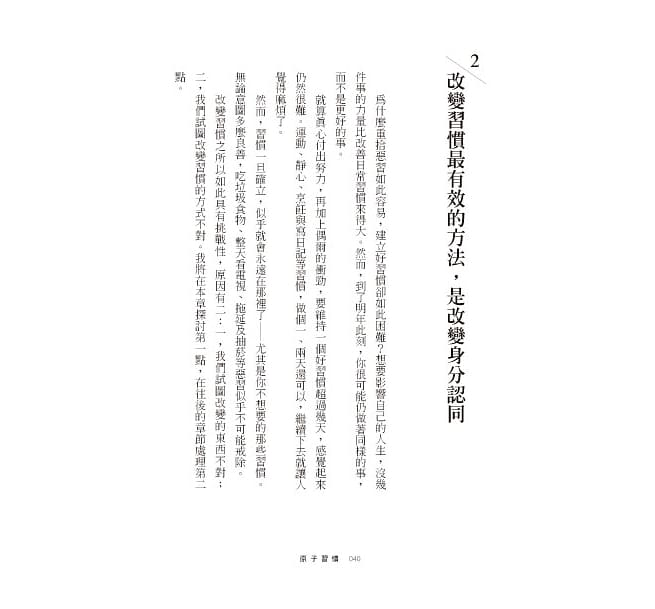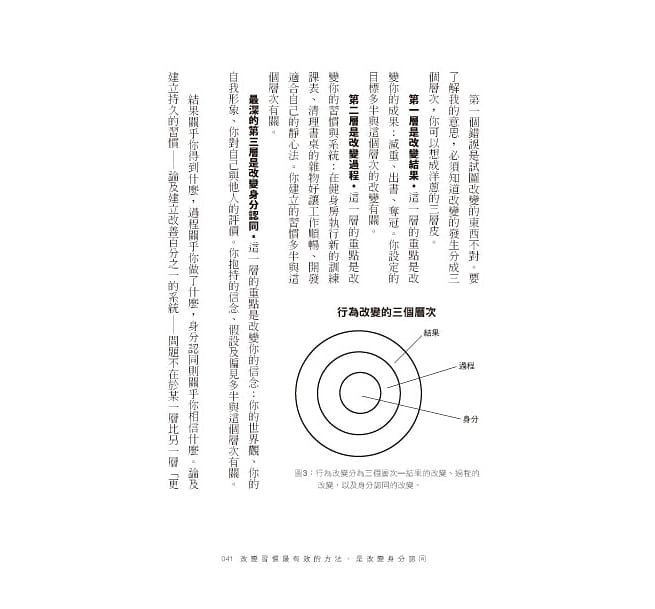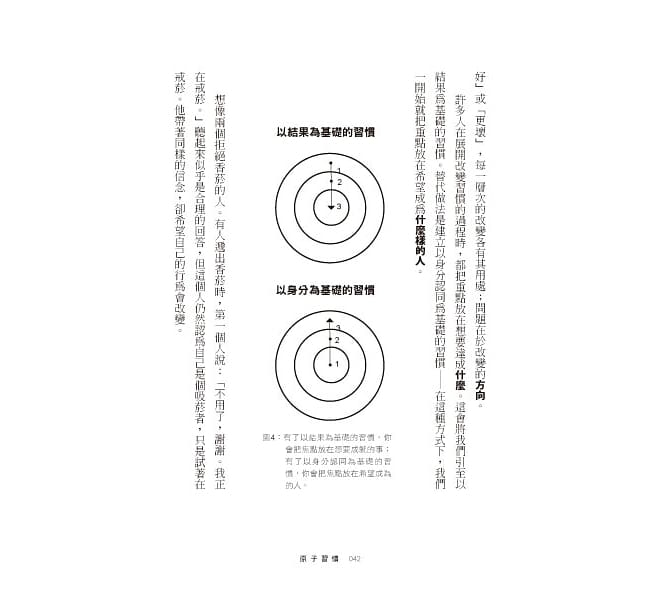## 擴展思考維度 - 心、技、體## 總結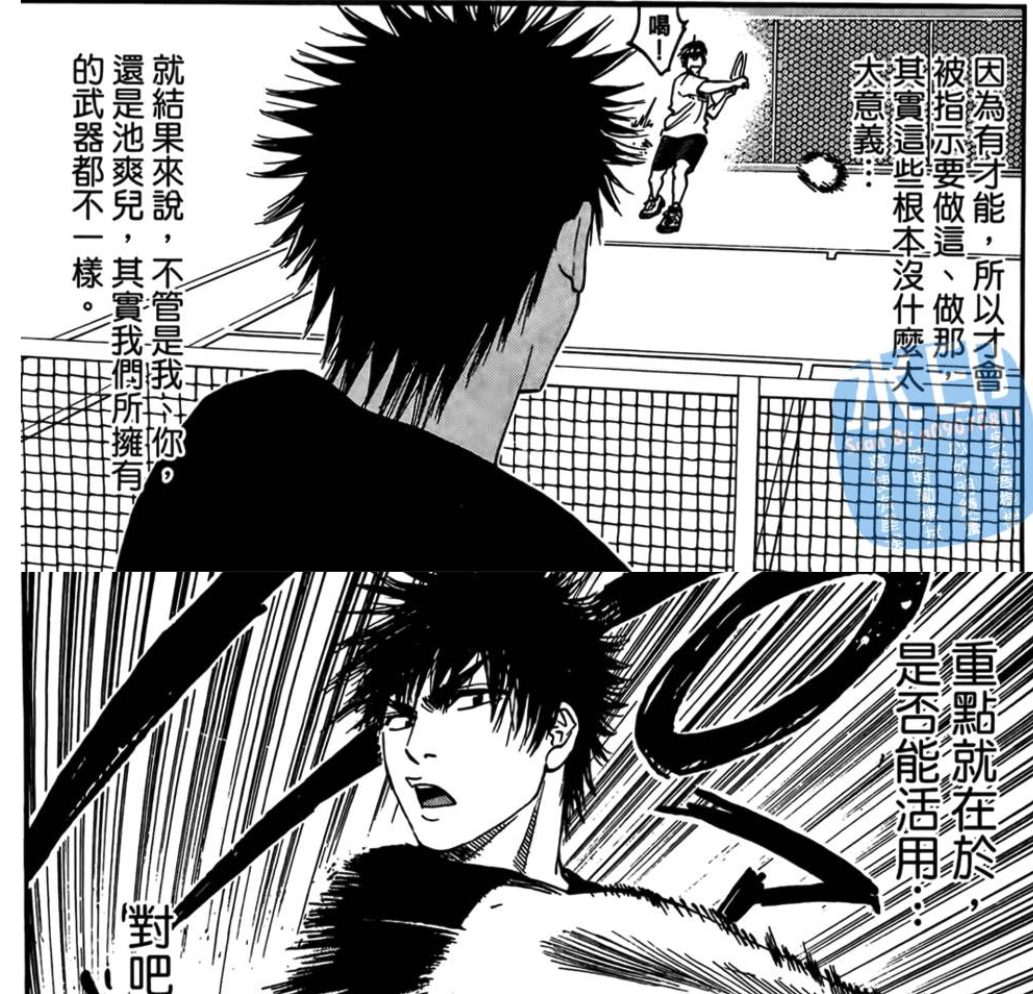## 延伸閱讀

#Self-improvement